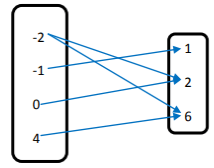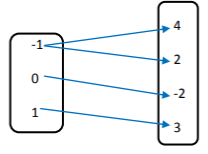# Relations and Mapping Worksheets | Worksheet on Relations and Functions with Solutions

If you need help on Relations and Mapping solve different questions from Relations and Mapping Worksheets. Try to solve various questions on Relations and Mapping and get the concepts underlying easily. The following sections include questions on Ordered Pairs, Cartesian Product of Two Sets, Identifying whether a Mapping Diagram is function or not, Representation of Math Relation, Domain, and Range, etc.

The Worksheets on Relations and Mapping include both complex and sample problems. Students can practice them and get step by step solutions to several example problems. The worksheet over here explains how to interpret and present relations as graphs, ordered pairs, arrow diagrams, etc.

1. Express the Relation as a Set of Ordered Pairs?Solution:

Ordered Pairs for the given relation

{(-2,2), (-2, 6), (-1,1) (0, 2) (4, 6)}

2. Function g(x) = 6x2+2x-2 find the value of g(-1)?

Solution:

g(x) = 6x2+2x-2

g(-1) = 6.(-1)2+2(-1)-2

= 6.1-2-2

= 6-4

= 2

3. Function f(x) is given by x3. Find the value of f(2)-f(1)/2-1?

Solution:

f(x) = x3

f(2) = 23
= 8
f(1) = 13
= 1
(f(2)-f(1))/2-1 = (8-1)/2-1

= 7/1

= 7

4. If If (4x + 2, 2y – 3) = (4, 3) find the values of x, y?

Solution:

Given (4x + 2, 2y – 3) = (4, 3)

As per equality of ordered pairs the first components and second components needs to be equal.

4x+2 = 4,  2y-3 = 3

4x= 4-2,  2y = 3+3

4x = 2,    2y = 6

x = 2/4,    y = 6/2

x = 1/2,   y = 3

Therefore, values of x, y are 1/2 and 3.

5. From the set of Ordered Pairs {(2, 8); (3, 9); (3, 5); (1, 7)} find the Domain and Range?

Solution:

From the set of ordered pairs given duplicates are not allowed for domain and range.

Domain = {2, 3, 1}

Range = {8, 9, 5, 7}

6. Following Figure Shows a Relationship from Set A to B. Write the Relation in Roster Form and also provide the Domain and Range?Solution:

From the above arrow diagram, we can write the relation from Set A to Set B in Roster Form as

R = {(-2, 4) (2, 4) (4, 16) (5, 25) (6, 36)}

Domain = { -2, 2, 4, 5, 6}

Range = {4, 16, 25, 36}

Repetitions are not allowed in the domain and range.

7. For the Relation given in Tabular Form draw the mapping diagram?Solution:

The above tabular form relation can be expressed in Mapping as such8. Let A = {3, 4, 5, 6} B = {x, y, z} find the Cartesian Product of AxB?

Solution:

Given A = {3, 4, 5, 6} B = {x, y, z}

AxB = {(3,x) (3, y) (3, z) (4, x) (4, y) (4, z) (5, x) (5, y) (5, z) (6, x) (6, y) (6, z)}

9. Write the Domain and Range for the following Relations?

(a) R₁ = {(6, 2); (6, 5); (3, 5); (0, 8); (7, 3)}
(b) R₂ = {(x, 2); (y, 3); (z, 2); (u, 6)}

Solution:

The domain is the first component of the ordered pairs and range is the second component of the ordered pairs. Repetitions are not allowed in both Domain and Range.

(a) R₁ = {(6, 2); (6, 5); (3, 5); (0, 8); (7, 3)}

Domain = { 6, 3, 0, 7}

Range = { 2, 5, 8, 3}

(b) R₂ = {(x, 2); (y, 3); (z, 2); (u, 6)}

Domain = { x, y, z, u}

Range = { 2, 3, 6}

10. If AxB = {(x, 3); (x, 4); (x, 5); (y, 3); (y, 4); (y, 5)}. Find BxA?

Solution:

AxB = {(x, 3); (x, 4); (x, 5); (y, 3); (y, 4); (y, 5)}

BxA = {(3, x) (4, x) (5, x) (3, y) (4, y) (5, y)}